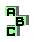PARRY REFLECTION POINT

 r138 point X(110), circumcenter r202 symmedian point, Fermat axis, point X(265) r328 isogonal points, cevian triangle, pedal triangle, similar triangles r427 incenter, excenters, concyclic points r507 circumcircle, point X(115), point X(110), Parry point, Parry circle, concyclic points r949 antigonal points, Lester circle r983 circlecevian triangle, orthocenter, circumcenter r1394 tangential triangle r1584 centroid, Euler line, point X(110) r1715 orthic triangle, Parry reflection point r1747 Fermat points, isodynamic points, Parry circle, point X(110), centroid, concyclic points r1764 antigonal points, medial triangle, antipedal triangle, circumcenter, perspective, homothetic triangles